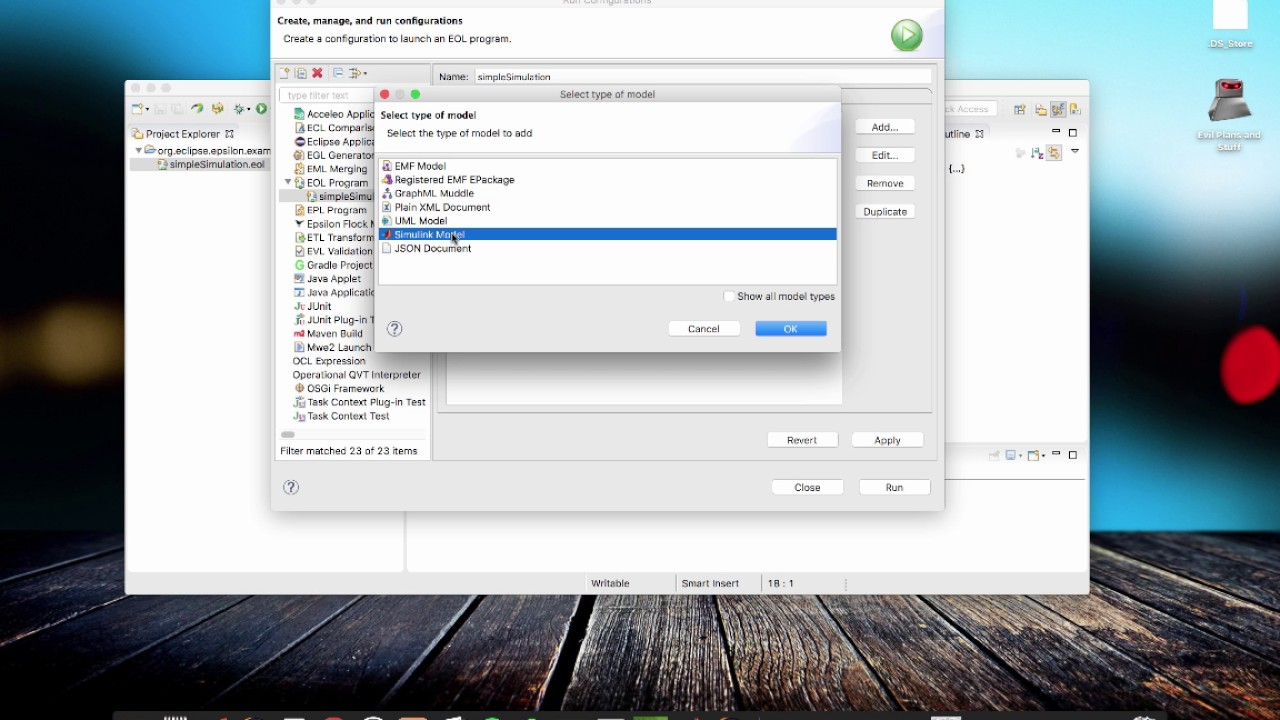# Scripting Simulink models using Epsilon

In this article we demonstrate how you can create, query and modify Simulink models in Epsilon programs. While the example in this article demonstrates using EOL to script Simulink models, it's worth stressing that Simulink models are supported throughout Epsilon. Therefore, you can use Epsilon to (cross-)validate, transform (to other models - Simulink or EMF-based -, or to text), compare and merge your Simulink models.## Installation

The first time you run Eclipse after installing the Simulink driver you will need to point it to the location of your Matlab installation through the Eclipse Preferences→Epsilon→Simulink dialog. In particular, you need to specify the location of the <matlab-root>/bin/win64 (or /bin/maci64 if you are on Mac OS) directory and Matlab's engine.jar as shown below. If you are on Windows, ensure that <matlab-root>/bin/win64 is in the system path.### Notes

• The driver has been developed and tested with Matlab_R2017a and may not work with older versions of Matlab (engine.jar was introduced fairly recently).
• If you start getting strange errors about dynamic libraries, chances are that the Matlab paths above are wrong. If restarting Eclipse and correcting the paths doesn't solve this, please post a message to the forum.

## Quick start

• Create a new general project
• Create an empty Simulink model in the project (e.g. empty.slx)
• Create a new EOL file (e.g. demo.eol) and add the content below to it:
```// Create elements
var sineWave = new `simulink/Sources/Sine Wave`;
var gain = new `simulink/Math Operations/Gain`;
var saturation = new `simulink/Discontinuities/Saturation`;
var busCreator = new `simulink/Signal Routing/Bus Creator`;
var scope = new `simulink/Sinks/Scope`;

// Position them on the diagram
sineWave.position = "[100 100 130 130]";
gain.position = "[200 100 230 130]";
saturation.position = "[300 100 330 130]";
busCreator.position = "[400 70 410 300]";
scope.position = "[500 175 530 205]";

// Set their properties
gain.gain = 2;
busCreator.inputs = 3;